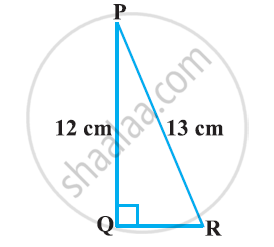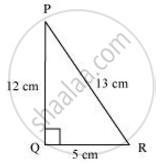# In Given Figure, Find tan P – cot R. - Mathematics

In Given Figure, find tan P – cot R.#### Solution

Applying Pythagoras theorem for ΔPQR, we obtain

PR2 = PQ2 + QR2

(13 cm)2 = (12 cm)2 + QR2

169 cm2 = 144 cm2 + QR2

25 cm2 = QR2

QR = 5 cmtan P = ("Side opposite to"angle P)/("Side adjacent to"angleP) = (QR)/(PQ)

= 5/12

cot R = ("Side opposite to"angle R)/("Side adjacent to"angleR) = (QR)/(PQ)

= 5/12

tan P - cot R =  5/12 - 5/12 = 0

Concept: Trigonometric Ratios
Is there an error in this question or solution?
Chapter 8: Introduction to Trigonometry - Exercise 8.1 [Page 181]

#### APPEARS IN

NCERT Class 10 Maths
Chapter 8 Introduction to Trigonometry
Exercise 8.1 | Q 2 | Page 181
Share